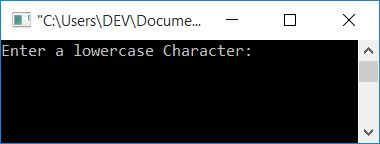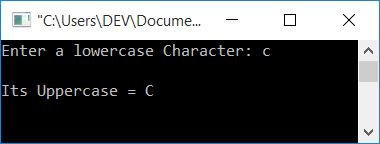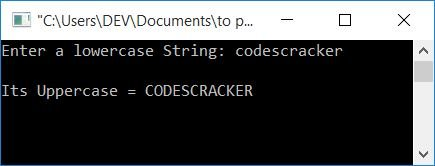# C Program to Convert Lowercase to Uppercase

In this article, you will learn and get code for converting any character or string from lowercase to uppercase. Here is the list of programs you will learn about:

But before going to these programs, let's first understand lowercase and uppercase characters, or strings.

### Lowercase Character

A character written in small letters is known as a lowercase character. For example, c.

### Lowercase String

If each and every character of a string written in small letters is known as a lowercase string. For example, codescracker.

### Uppercase Character

A character written in capital letters is known as an uppercase character. For example, C.

### Uppercase String

If each and every character of a string is written in capital letter, the string is known as an uppercase string. For example, CODESCRACKER.

### All Alphabets' ASCII Value

ASCII values of A-Z (uppercase alphabets) are 65-90. That is, the ASCII value of

• A is 65
• B is 66
• C is 67
• and so on.

Letters a-z (lowercase alphabets) have ASCII values 97-122. That is, the ASCII value of

• a is 97
• b is 98
• c is 99
• and so on.

### Lowercase to Uppercase Formula

As you can see, the ASCII values of lowercase and uppercase alphabets are given above. The lowercase alphabet's ASCII value, say, "a," is 32 greater than the uppercase alphabet's ASCII value, say, "A." So to convert lowercase to uppercase, use the formula

`upperChar = lowerChar-32`

Where lowerChar is the character entered by the user (in lowercase). For example, if lowerChar contains a, then using the above formula

```upperChar = lowerChar-32
=a-32
=97-32
=65```

And 65 is the ASCII value of A. Therefore, the given lowercase character a gets converted into an uppercase character A. To apply the conversion to a string, convert each and every character using the above formula. Now let's move on to the program given below:

## Lowercase to Uppercase Character in C

Let's first create a program that converts lowercase characters to uppercase characters. So the question is: write a program in C that asks the user to enter a character (in lowercase) and converts it to uppercase. The answer to this question is given below:

```#include<stdio.h>
#include<conio.h>
int main()
{
char lowerChar, upperChar;
int ascii;
printf("Enter a lowercase Character: ");
scanf("%c", &lowerChar);
ascii = lowerChar;
upperChar = ascii-32;
printf("\nIts Uppercase = %c", upperChar);
getch();
return 0;
}```

This program was built and runs under the Code::Blocks IDE. Here is the sample run:Now supply any character (in lowercase) as input, say "c," and press the ENTER key to see the following output:## Lowercase string to uppercase in C

The question is: write a program in C that converts any given string from lowercase to uppercase. The answer to this question is given below:

```#include<stdio.h>
#include<conio.h>
int main()
{
char lowerString="", upperString="";
int i=0;
printf("Enter a lowercase String: ");
gets(lowerString);
while(lowerString[i]!='\0')
{
upperString[i] = lowerString[i]-32;
i++;
}
printf("\nIts Uppercase = %s", upperString);
getch();
return 0;
}```

The snapshot given below shows the sample run of this program:As you can see from the above program, each and every character gets converted into its equivalent value in uppercase inside the while loop. After converting, just print the value of upperString as output.

## Using a Library Function to convert a lowercase string to an uppercase string in C

This is the end of this article. This program uses the standard library function strupr(), which takes a string as an argument and returns the string given in the argument in its uppercase form. Let's have a look at the program:

```#include<stdio.h>
#include<conio.h>
#include<string.h>
int main()
{
char str;
printf("Enter lowercase String: ");
gets(str);
printf("\nIts Uppercase = %s", strupr(str));
getch();
return 0;
}```

The output produced by this program is the same as that of the previous program.

#### The same program in different languages

C Quiz

« Previous Program Next Program »